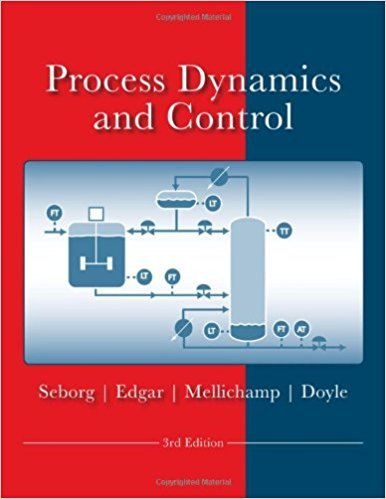×
Get Full Access to Process Dynamics And Control - 3 Edition - Chapter 6 - Problem 6.6
Get Full Access to Process Dynamics And Control - 3 Edition - Chapter 6 - Problem 6.6

×

# A process consists of an integrating element operating inISBN: 9780470128671 148

## Solution for problem 6.6 Chapter 6

Process Dynamics and Control | 3rd Edition

• Textbook Solutions
• 2901 Step-by-step solutions solved by professors and subject experts
• Get 24/7 help from StudySoup virtual teaching assistantsProcess Dynamics and Control | 3rd Edition

4 5 1 430 Reviews
31
2
Problem 6.6

A process consists of an integrating element operating in parallel with a first-order element (Fig. E6.6). U(s) Y(s) Figure E6.6 (a) What is the order of the overall transfer function, G(s) = Y(s)/U(s)? (b) What is the gain of G(s)? (c) What are the poles of G(s)? Where are they located in the complex s-plane? (d) What are the zeros of G(s)? Where are they located? Under what condition(s) will one or more of the zeros be located in the right-half s-plane? (e) Under what conditions, will this process exhibit a righthalf plane zero? (f) For any input change, what functions of time (response modes) will be included in the response, y(t)? (g) Is the output bounded for any bounded input change, for example, u(t) = M?

Step-by-Step Solution:

Problem 6.6A process consists of an integrating element operating in parallel with a first-order element(Fig. E6.6). U(s) Y(s) Figure E6.6(a) What is the order of the overall transfer function, G(s) = Y(s)/U(s)(b) What is the gain of G(s)(c) What are the poles of G(s) Where are they located in the complex s-plane(d) What are the zeros of G(s) Where are they located Under what condition(s) will one ormore of the zeros be located in the right-half s-plane(e) Under what conditions, will this process exhibit a right half plane zero(f) For any input change, what functions of time (response modes) will be included in theresponse, y(t)(g) Is the output bounded for any bounded input change, for example, u(t) = M Step-by-step solution Step 1 of 9 ^(a)Refer to Figure E6.6 in the textbook for the closed-loop transfer function. K 1 K2 Y (s) = s U(s) + s + 1U(s) K1 K2 Y (s) = ( s + s + 1 U(s) Y (s) K K = s1+ s + 1 U(s) K (s+1)+K s Y (s)= 1 2 U(s) s(s+1) Y (s) K1(s+1)+K 2 U(s) = (s +s) (K +K )s+K Y (s)= 1 2 1 U(s) (s +s) Y (s)Substitute G(s) for U(s) in the above equation. (K +K )s+K G(s) = 1 2 1 ……….(1) (s +s)From equation (1), the maximum exponent on s in denominator is 2.\nTherefore, the order of overall transfer function is .

Step 2 of 9

Step 3 of 9

##### ISBN: 9780470128671

The answer to “A process consists of an integrating element operating in parallel with a first-order element (Fig. E6.6). U(s) Y(s) Figure E6.6 (a) What is the order of the overall transfer function, G(s) = Y(s)/U(s)? (b) What is the gain of G(s)? (c) What are the poles of G(s)? Where are they located in the complex s-plane? (d) What are the zeros of G(s)? Where are they located? Under what condition(s) will one or more of the zeros be located in the right-half s-plane? (e) Under what conditions, will this process exhibit a righthalf plane zero? (f) For any input change, what functions of time (response modes) will be included in the response, y(t)? (g) Is the output bounded for any bounded input change, for example, u(t) = M?” is broken down into a number of easy to follow steps, and 127 words. Since the solution to 6.6 from 6 chapter was answered, more than 455 students have viewed the full step-by-step answer. The full step-by-step solution to problem: 6.6 from chapter: 6 was answered by , our top Engineering and Tech solution expert on 11/15/17, 04:03PM. Process Dynamics and Control was written by and is associated to the ISBN: 9780470128671. This textbook survival guide was created for the textbook: Process Dynamics and Control, edition: 3. This full solution covers the following key subjects: Plane, located, process, Where, under. This expansive textbook survival guide covers 24 chapters, and 420 solutions.

Unlock Textbook Solution